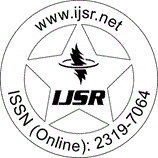International Journal of Science and Research (IJSR)
Call for Papers | Fully Refereed | Open Access | Double Blind Peer Reviewed

Research Paper | Electrical Engineering | India | Volume 6 Issue 3, March 2017

# Modeling and Simulation of Matrix Converter Using Space Vector PWM Technique

O. Hemakesavulu | T. Brahmananda Reddy

Abstract: Matrix Converters can directly convert an ac power supply of fixed voltage into an ac voltage of variable amplitude and frequency. Matrix Converter is a single stage converter. The matrix converters has following advantages over conventional converters with low volume, sinusoidal input current, bidirectional power flow, minimizing of lower order harmonics and lack of bulky reactive elements. All the reasons lead to the development of matrix converter. Based on the control techniques used in the matrix converter, the performance varies. So this paper analyses the performance of matrix converter with two different modulation techniques such as PWM and SVPWM. The basic principle and switching sequence of these modulation techniques are presented in this paper. The output voltage, output current waveforms and THD spectrum of switching waveforms connected to RL load are analyzed by using Matlab/Simulink software. The simulated results are analyzed and show that the THD is better for SVPWM technique

Keywords: Matrix Converter, Pulse Width Modulation PWM, Space Vector Pulse Width Modulation SVPWM, Total Harmonic Distortion THD

Edition: Volume 6 Issue 3, March 2017,

Pages: 1754 - 1758